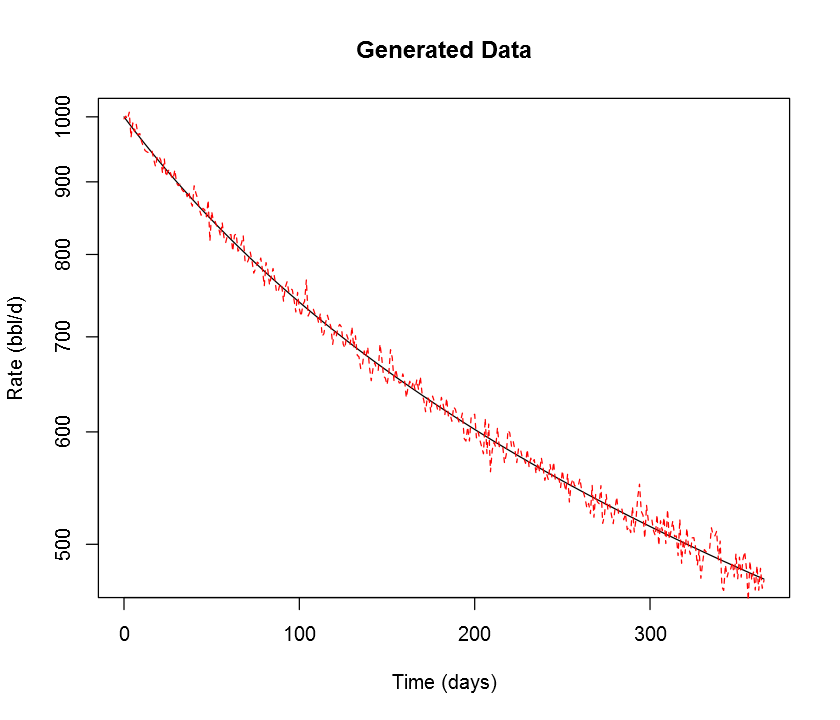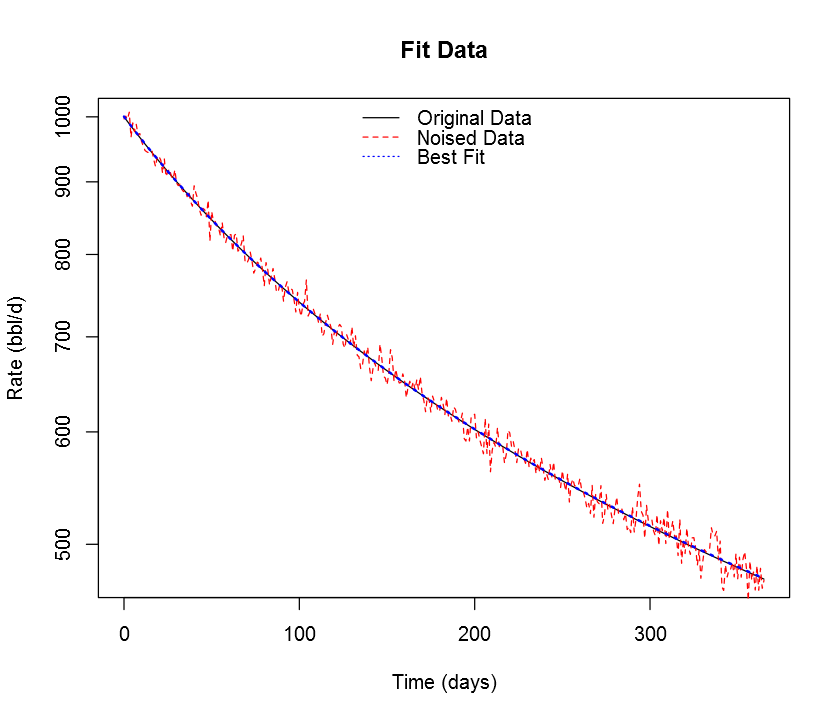## aRpsDCA examples¶

aRpsDCA isn't picky about data formats; all the functions work in terms of simple vectors of time and rate or volume data. We also provide some functions for unit conversion.

For example, let's generate some data following a hyperbolic decline, add some noise, and try to recover it using the best fit function.

First, we'll need to import the package.

In :
require(aRpsDCA)


I also have to toggle graphics mode to work around a bug in the R kernel for IPython; you won't need to worry about this.

I'm also going to make the plots a little smaller than default.

In :
options(jupyter.plot_mimetypes = 'image/png')
options(repr.plot.width=7, repr.plot.height=6)


Now, let's generate some data following a hyperbolic decline with $q_i$ = 1000 bbl/d, $D_i$ = 75% tangent effective per year (note that many DCA packages present units in terms of secant effective decline: these can be converted following the equations in SPEE monograph #3, available online), and $b$ = 1.5.

In :
t <- seq(0, 365) # one year, in days
q <- hyperbolic.q(
qi=1000, # units must match for rate, time, decline
# we use helper functions from aRpsDCA to handle
# effective -> nominal conversion and change units
Di=as.nominal(0.75, from.period="year", to.period="day"),
b=1.5,
t)
plot(q ~ t, log="y",
main="Generated Data", xlab="Time (days)", ylab="Rate (bbl/d)",
type="l")Ok, let's add some random (Gaussian) noise to make this a nice example data set to try to fit. We'll see how close we come to recovering the original parameters.

I'm setting a fixed seed so this example comes out the same every time.

In :
set.seed(555)

q.noised <- q + rnorm(length(q), mean=0, sd=10)
plot(q ~ t, log="y",
main="Generated Data", xlab="Time (days)", ylab="Rate (bbl/d)",
type="l")
lines(q.noised ~ t, col="red", lty="dashed")Now, let's see how well we can recover the curve parameters with the best hyperbolic fit function.

In :
# to be honest, I am not sure where the vapply(...) error comes from below;
# I can't reproduce it outside jupyter and it doesn't alter the result, so
# I'm chalking it up to some issue in the IR kernel.

fit.curve <- best.hyperbolic(q.noised, t)
fit.curve

Error in vapply(seq_along(mapped), function(i) {: values must be length 1,
but FUN(X[]) result is length 0

Out:
$decline  "Arps hyperbolic decline: <qi = 1000.598, Di = 0.003811069, b = 1.512116>"$sse
 37651.8


The object-oriented design of aRpsDCA also lets us apply this fit result directly against the time data. Note that the printed $q_i$ and $D_i$ values are in daily units (nominal daily for $D_i$).

In :
fit.forecast <- arps.q(fit.curve$decline, t) plot(q ~ t, log="y", main="Fit Data", xlab="Time (days)", ylab="Rate (bbl/d)", type="l") lines(q.noised ~ t, col="red", lty="dashed") lines(fit.forecast ~ t, col="blue", lty="dotted", lwd=2) legend("top", lty=c("solid", "dashed", "dotted"), col=c("black", "red", "blue"), bty="n", legend=c("Original Data", "Noised Data", "Best Fit"))Finally, we'll convert the best fit$D_i$value back to a yearly tangent effective rate to compare to the original. In : as.effective(fit.curve$decline\$Di, from.period="day", to.period="year")

Out:
0.751420575465941

Pretty close!